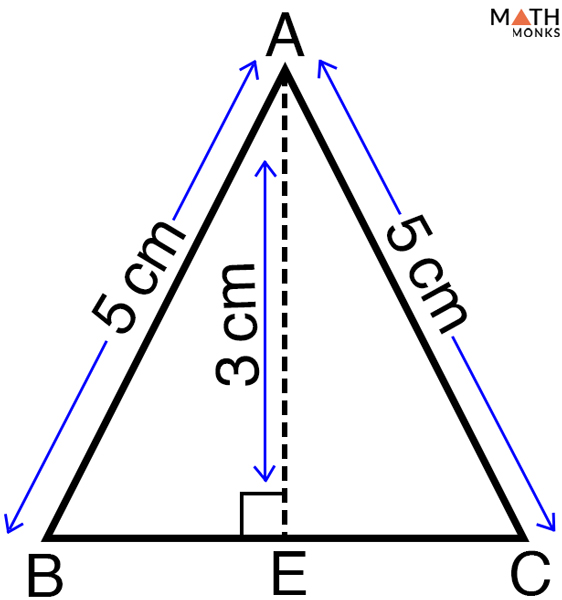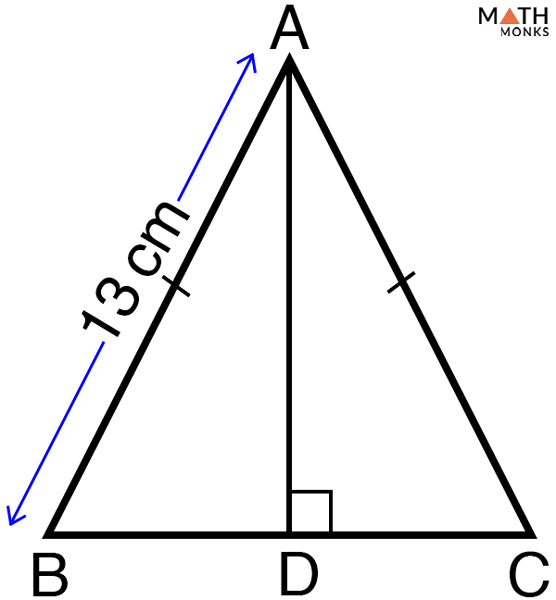# Base of a Triangle

## Definition

Base meaning bottom, it refers to any side of a triangle, which is perpendicular to its height or altitude. For scalene or equilateral triangle, any side can be the base, while for an isosceles triangle it is the single, unequal side.

## Formula

The formula for base of a triangle can be derived from the standard formula of area of a triangle as shown below:

As we know,

Area (A) = ½ (b x h), here b = base, h = height

=> 2A = b x h

=> b = 2A/h

Hence, mathematically, base of a triangle can also be defined as twice the area divided by the height of the triangle.

## How to Find the Base of a Triangle

Let us solve some problems to derive the base of the given triangles.

Problem: Finding the base of a triangle, when the HEIGHT and the AREA are known.

Find the base of a triangle with area 12 cm2 and height 8 cm.

Solution:

As we know,
b = 2A/h, here area = 12cm2, h = 8 cm
= 2 x 12/8
= 3 cm

Problem: Finding the base of a right triangle, when the HEIGHT and the HYPOTENUSE are known.

Identify the base of a right triangle having height 6 cm and hypotenuse 9 cm.

Solution:

Here, we will use the Pythagorean Theorem,
(Hypotenuse)2 = (Base)2 + (Height)2, here height = 6 cm and hypotenuse = 9 cm
(6) 2 = (b)2 + (3)2
(b)2 = (6) 2 – (3)2
b2 = 36- 9
b = 25 = 5 cm.

Problem: Finding the base of an isosceles triangle, when the SIDES and the HEIGHT are known.Find the missing base of the given isosceles triangle with two sides measuring 5 cm each and having height of 3 cm.

Solution:

Here, we will use the Pythagorean Theorem,
In △ABC,
Since, the height AE divides the △ABC into two similar right triangles, △ABE and △ACE and the base BC into BE and EC equally
Such that,
BC = BE + EC
Now,
According to the Pythagoras theorem,
(Hypotenuse)2 = (Base)2 + (Height)2
In △ABE,
(AB)2 = (BE)2 + (AE)2, here AB = 3 cm, AE = 5 cm
(BE)2= (AB)2 – (AE)2
(BE)2 = (5) 2 – (3)2
(BE)2 = 25 – 9
(BE)2 = 16
BE = 4 cm
Similarly, EC = 4 cm
Thus,
BC = BE + EC = 8 cmFind the base of the given isosceles triangle with an area of 60 cm2 and the length of one of the equal sides is 13 cm.

Solution:

Let the base = 2x and height = h
As we know,
Area of △ABC = ½ (b x h) = 60 cm2
⇒½(b x h) = 60, here b = 2x
⇒ ½ x 2x x h = 60
⇒ xh = 60 …… (1)
Now, using Pythagorean theorem in △ABD, we get
132 = h2 + x2
⇒ h2 + x2 = 169
Adding 2hx on both sides we get,
⇒ h2 + x2 + 2hx = 169 + 2hx
⇒ (h + x)2 = 169 + 2 x 60
⇒ (h + x)2 = 289
⇒ h + x = 17
⇒ h = 17 – x …… (2)
Substituting (2) in (1), we get,
x (17 – x) = 60
17x – x2 = 60
⇒ -x2 + 17x – 60 = 0
⇒ x2– 17x + 60 = 0
⇒ x2– 12x – 5x +60 = 0
⇒ x(x-12) – 5(x + 60)  =0
⇒ (x-12) (x – 5) = 0
Either,
x -12 = 0
x = 12
Or,
x – 5 =0
x = 5
Hence, base of the given isosceles triangle is,
b = 2x = 2 x 5 =10 cm (if x = 5) or, 2x = 2 x 12 = 24 cm (if x = 12)